### Classification Of Elements- The Periodic Table Class 10th Physics & Chemistry AP Board Solution

##### Question 1.Newlands proposal the law of octaves. Mendeleeff suggested eight groups for elements in his table. How do you explain these observations in terms of modern periodic classification?Answer:Law of octaves in terms of modern periodic tablei. Law of octaves states that “every eighth element has properties similar to the firsts element”ii. In modern periodic table, any element of the same period has similar properties.iii. For example-Mg and Ca exhibits similar properties as they both belong to same group.Mendleeff periodic tablei. He suggested eight groups for elements in his table.ii. In modern periodic table, the vertical columns are called groups. There are total 18 groups present.Question 2.What are the limitations of Mendeleeff’s periodic table? How could the modern periodic table overcome the limitations of Mendeleeff’s table?Answer:Limitations of Mendleeff’s periodic table are:i. Certain elements of highest atomic weight precede those with lower atomic weight. For example – Tellurium (atomic weight 127.6) precedes iodine (atomic weight 126.9)ii. Elements having different properties were placed in the same group.iii. Isotopes were discovered long time after Mendeleev put forth the periodic table. As isotopes have the same chemical properties but different atomic masses, a challenge was posed in placing them in Mendeleev’s periodic table.The modern periodic table overcome the limitations of Mendeleeff’s table:i. Moseley realized that atomic number is the fundamental property of an element than its weight.ii. He arranged the elements in the periodic table according to their atomic numbers.iii. This arrangement removes the problem of atomic weights.iv. In the modern periodic table, isotopes are put together with their elements.Question 3.Define the modem periodic law. Discuss the construction of the long form of the periodic table.Answer:Modern periodic law states that “the physical and chemical properties of elements are the periodic function of their electronic configurations of their atoms”.i. The modern periodic table contains horizontal periods 1 to 7.ii. Similarly, it contains vertical groups 1 to 18.iii. Atomic numbers are indicated on the upper part of the element.iv. Two rows are separately placed at the bottom of the periodic table. These are called lanthanide series and actinoid series.v. There are 118 boxes in the periodic table.vi. The whole periodic table is divided into four blocks.vii. The left side is s-block, right side is p-block, in the middle there is d-block and the lanthanide series and actinoid series form the f-block.viii. The first period contains 2 elements.ix. The s-block contains alkali and alkaline earth metals.x. The p-block contains metals, non-metals and metalloids.xi. The d-block contains transition metals.Question 4.Explain how the elements are classified into s, p, d and f- block elements in the periodic table and give the advantage of this kind of classification.Answer:Classification of elementsi. The s-block contains alkali metals (period 1) and alkaline earth metals (period 2).ii. The p-block (period 13- 18) contains metals, non- metals and metalloids.iii. The d-block (period 3-12) contains transition metals.iv. The f-block placed separately at the bottom of the table contains lanthanides and actinides.Question 5.Given below is the electronic configuration of elements A, B, C, D.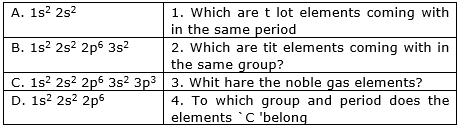Answer:a. A and B are the elements coming within the same period.i. As the total no of valence electrons (electrons in the outermost shell) decides the period number of the element.ii. A (1s2 2s2) and B (1s2 2s2 2p6 3s2) both have two valence electrons.Hence, they belong the same period, i.e., second period.b. B and C are the elements coming within the same group.⇒ As the highest principal quantum(n) number decides the group number of the element.⇒ In B (1s2 2s2 2p6 3s2) and C (1s2 2s2 2p6 3s2 3p3), 3 is the highest quantum number.⇒ Hence B and C belong to the same group, i.e., third groupc. D is the noble gas element⇒ D has atomic number 10.⇒ This means 2 in K-shell and 8 in the L-shell which is a completeNoble gas configuration.d. C belongs to 5th period and 3rd group.⇒ C has 5 electrons in the outermost shell (3s2 3p3). Hence it belongs to 5th period.⇒ In C (1s2 2s2 2p6 3s2 3p3), 3 is the highest quantum number. Hence, it belongs to third period.Question 6.Write down the characteristics of the elements having atomic number 17.Electronic configuration ………………………….Period number ………………………….Group number ………………………….Element family ………………………….No. of valence electrons ………………………….Valency ………………………….Metal or non-Metal ………………………….Answer:a. The electronic configuration of the element (Z=17) is:1s2 2s2 2p6 3s2 3p5b. Period number = 7As the element has 7 electrons in the outermost shell (3s2 3p5). Hence, it belongs to period 7.c. Group number = 3In the element (1s2 2s2 2p6 3s2 3p5), 3 is the highest principal quantum number. Hence, it belongs to third period.d. Element family = p-block elementThe element belongs to group 3 and period 7, this means it is located at the right side of the periodic table. Hence, it belongs to p-block family.e. Valence electrons = 7The element (1s2 2s2 2p6 3s2 3p5) has 7 valence electrons, i.e., electrons in the outermost shell (3s2 3p5).f. Valency = 1The valency is the number of electrons needed to complete the octet. So the element (2,8,7) need only one electron to achieve noble gas configuration (2,8,8)g. Non-metalQuestion 7.State the number of valence electrons, the group number and the period number of each element given in the following table: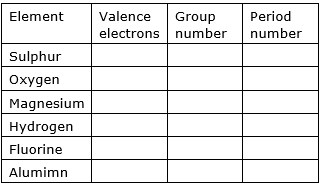Answer: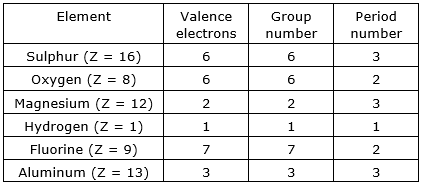SulphurThe atomic number of sulphur = 16The electronic configuration of 16S = 1s2 2s2 2p6 3s2 3p4⇒ Total number of valence electrons (3s2 3p4) = 6⇒ As period number is decided by the valence electrons, thus period number of sulphur = 6⇒ In sulphur, 3 is the highest principal quantum number (n=3), thus group number of sulphur = 3OxygenThe atomic number of oxygen = 8The electronic configuration of 8O = 1s2 2s2 2p4⇒ Total number of valence electrons (2s2 2p4) = 6⇒ As period number is decided by the valence electrons, thus period number of oxygen = 6⇒ In oxygen, 2 is the highest principal quantum number (n=2), thus group number of oxygen = 2MagnesiumThe atomic number of magnesium = 12The electronic configuration of 12Mg = 1s2 2s2 2p6 3s2⇒ Total number of valence electrons (3s2) = 2⇒ As period number is decided by the valence electrons, thus period number of magnesium = 2⇒ In magnesium, 3 is the highest principal quantum number (n=3), thus group number of magnesium = 3HydrogenThe atomic number of hydrogen = 1The electronic configuration of 1H = 1s1⇒ Total number of valence electrons (1s1) = 1⇒ As period number is decided by the valence electrons, thus period number of hydrogen = 1⇒ In hydrogen, 1 is the highest principal quantum number (n=1), thus group number = 1FluorineThe atomic number of fluorine = 9The electronic configuration of 9F = 1s2 2s2 2p5⇒ Total number of valence electrons (2s2 2p5) = 7⇒ As period number is decided by the valence electrons, thus period number of fluorine = 7⇒ In fluorine, 2 is the highest principal quantum number (n=2), thus group number of fluorine = 2AluminumThe atomic number of aluminum = 13The electronic configuration of 13Al = 1s2 2s2 2p6 3s2 3p1⇒ Total number of valence electrons (3s2 3p1) = 3⇒ As period number is decided by the valence electrons, thus period number of aluminum = 3⇒ In aluminum, 3 is the highest principal quantum number (n=3), thus group number of aluminum = 3Question 8.State whether the following elements belong to a Group (G), Period (P) or Neither Group nor Period (N).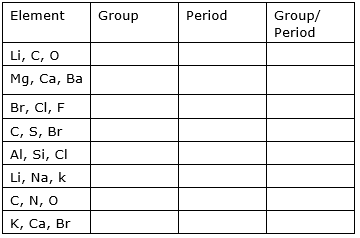Answer: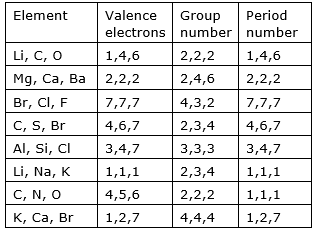In Li, C, O: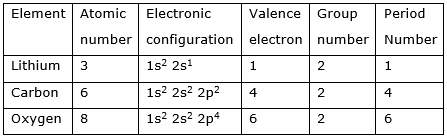All of three elements belong to same group, i.e., second groupIn Mg, Ca, Ba: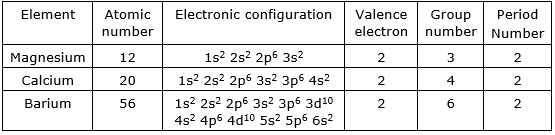All of three elements belong to same period, i.e., second periodIn Br, Cl, F: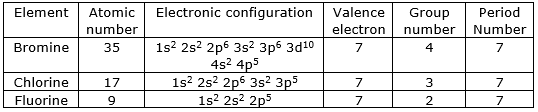All of three elements belong to same period, i.e., 7th periodIn C, S, Br: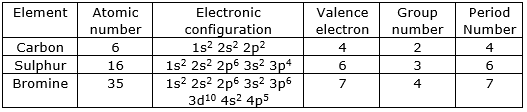All of three elements do not belong to same period or groupIn Al, Si, Cl: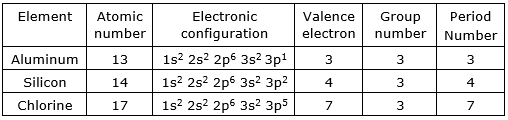All of three elements belong to same group, i.e., third groupIn Li, Na, K: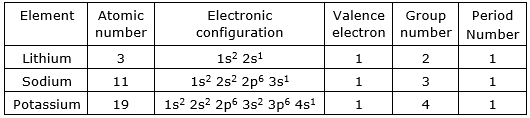All of three elements belong to same period, i.e., first periodIn C, N, O: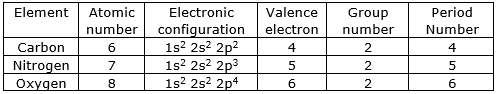All of three elements belong to same group, i.e., second groupIn K, Ca, Br: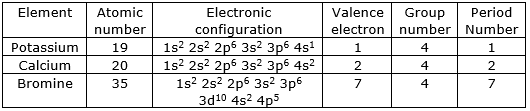All of three elements belong to same group, i.e., 4th groupQuestion 9.Elements in a group generally possess similar properties, but elements along a period have different properties. How do you explain this statement?Answer:Elements along a period possess different properties because:i. As we from left to right along a period, elements increases by one-unit charge between any two successive elements with increase in atomic number.ii. Thus, the electronic configuration of valence shell of any element in a given period is not same.iii. As a result, elements along a period have different properties.Question 10.s - block and p - block elements except 18th group elements are sometimes called as `Representative elements' based on their abundant availability in the nature. Is it justified? Why?Answer: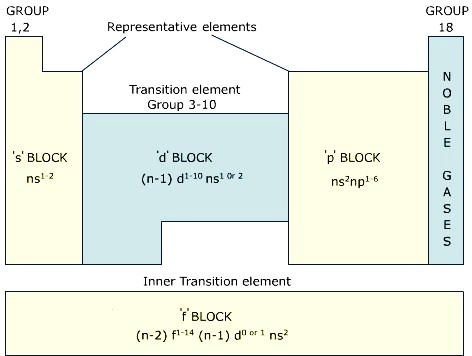The elements of s and p-block except noble gas are called as representative elements because:i. The outer shells of the elements of s and p-block are not completely filled.ii. They achieve noble gas configuration by losing or gaining electrons.iii. As a result, they are chemically inert active.iv. The elements down the group represent have same number of electrons in the outermost shell.v. They have same electronic configuration.vi. Hence, the s and p-block elements are called representative elements.Question 11.Complete the following table using the periodic table.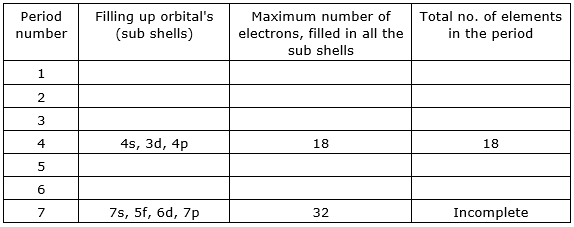Answer: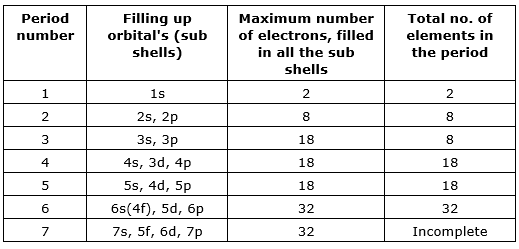Question 12.Complete the following table using the periodic table.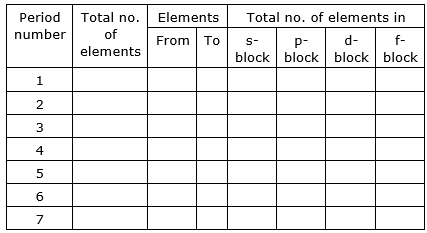Answer: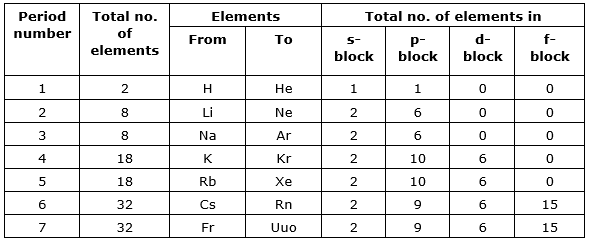Question 13.The electronic configuration of elements X, Y and Z are given below?a) X = 2b) Y = 2, 6c) Z= 2, 8, 2i) Which element belongs to second period?ii) Which element belongs in second group?iii) Which element belongs to 18th group?Answer:i) The element ‘Z’ belongs to second periodExplanation:As number of valence electrons decide the period of the element.⇒ Element ‘X’ has not any valence electrons.⇒ Element ‘Y’ has 6 valence electrons.⇒ Element ‘Z’ has 2 valence electrons.Thus, element ‘Z’ belongs to second period.ii) The element ‘Y’ belongs to second group.Explanation:As total number of shells present in the element decide the group number.⇒ Element ‘X’ has total one shell (2).⇒ Element ‘Y’ has total two shells (2,6).⇒ Element ‘Z’ has total three shells (2.8,2).The element ‘Y’ belongs to second group.iii) The element ‘X’ belongs to 18h group.Explanation:Element ‘X’ belongs to 18th group because it has complete noble gas configuration (X=2). This means it is a noble gas which are placed in 18th group.Question 14.Identify the element that has the larger atomic radius in each pair of the following and mark it with a symbol (3).(i) Mg or Ca(ii) Li or Cs(iii) N or P(iv) B or AlAnswer:Note: Atomic radius goes on decreasing as we move from left to right.Atomic radius goes on increasing from top to bottom.i) Ca has larger atomic radius because as we move from Mg to Ca, the atomic radius increases.ii) Cs has larger atomic radius because as we move from Li to Cs, the atomic radius increases.iii) P has larger atomic radius because as we move from N to P, the atomic radius increases.iv) Al has larger atomic radius because as we move from B to Al, the atomic radius increases.Question 15.Identify the element that has the lower Ionization energy in each pair oldie following and mark it with a symbol (3).(i) Mg or Na(ii) Li or O(iii) Br or F(iv) K or BrAnswer:Note: Ionization goes on increasing as we move from left to right.Ionization goes on decreasing from top to bottom.i) Na has lower ionization energy because as we move from Na to Mg, the ionization energy increases.ii) Li has lower ionization energy because as we move from Li to O, the ionization energy increases.iii) F has lower ionization energy because as we move from F to Br, the ionization energy decreases.iv) K has lower ionization energy because as we move from K to Br, the ionization energy increases.Question 16.In period 2, element X is to the right of element Y. Then, find which of the elements have:(i) Low nuclear charge(ii) Low atomic size(iii) High ionization energy(iv) High electro negativity(v) More metallic characterAnswer:i) Y has low nuclear chargea) The nuclear charge increases from left to right in a period because the atomic number increases.b) The outer electrons are added in the same valence shell.c) As a result, the attraction on the electrons by the nucleus increases as we move from left to right.d) Thus, Y has low nuclear charge.ii) X has low atomic size.a) Atomic radius goes on decreasing as we move from left to right.b) This is due to increase in attraction by the nucleus on the electrons.c) This tends to decrease the size with increase in atomic number.d) Thus, X has low nuclear charge.iii) X has higher ionization energy.Ionization goes on increasing as we move from left to right due to increased nuclear charge. Thus, X has higher ionization energy.Note: More is the nuclear charge; more is the ionization energy.iv) X has higher electronegativity.Electronegativity goes on increasing as we move from left to right due to increase in attraction between the outer electrons and the nucleus. Thus, X has higher electronegativity.v) Y has more metallic characterMetallic character goes on decreasing while going from left to right in a period due to increased attraction by the nucleus. Thus, Y has ore metallic character.Note: More easily an element loses electrons, higher will be its metallic character (electropositive character)Question 17.How does metallic character change when we move(I) Down a group(II) Across a period?Answer:Metals have a tendency to lose their valence electrons to form a cation.(I)Down a groupi. As we move from left to right, a new shell gets added.ii. The distance between the nucleus and the outer electrons.iii. As a result, nuclear charge decreases with increase in atomic number.iv. This decreases the force of attraction of electrons by the nucleus.v. As a result, the tendency of losing electrons goes on increasing.vi. Thus, metallic character increases within a group.(II) Across a periodi. As we move from left to right, nuclear charge increases with increase in atomic number. This increase the force of attraction of electrons by the nucleus.ii. As a result, the tendency of losing electrons goes on decreasing.iii. Thus, metallic character decreases within a period.Question 18.Why was the basis of classification of elements changed from the atomic mass to the atomic number?Answer:The basis of classification of elements changed from the atomic mass to the atomic number is:i. Certain elements of height atomic weight precede those with lower atomic weights.For example: tellurium precedes iodineii. After knowing atomic numbers of elements, it was recognized that a better way of arranging the elements in the periodic table is according to increasing g atomic number.iii. The periodic law is changed from atomic weight concepts to atomic number concepts.Question 19.What is a periodic property? How do the following properties change in a group and period? Explain.(a) Atomic radius(b) Ionization energy(c) Electron affinity(d) Electro negativity.(b) Explain the ionization energy order in the following sets of elements:a) Na, Al, Cl b) Li, Be, Bc) C N, O d) F, Ne, Na c) Be, Mg, CaAnswer:Periodic property: The modern periodic table is organized on the basis of electronic configuration of the atoms of elements.Physical and chemical property of elements are related to their electronic configurations particularly the outer shell configuration.a. Atomic radiusAtomic radius goes on decreasing while going from left to right in a period because of the following reasons:i. Within a period, the atomic number increases one by one as a result nuclear charge increases.ii. The outer electrons are adding in the same valence shell.iii. Due to increased nuclear charge, the attraction of electrons by the nucleus increases.iv. Therefore, the size of the atom decreases with the increase in atomic number (number of protons).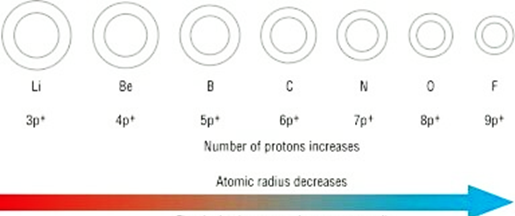Atomic radius goes on increasing down a group because:i. Down a group, the atomic number increases one by one as a result nuclear charge increases.ii. The outer electrons are adding in a new valence shell.iii. Therefore, the distance between the outermost electron (valence electron) and the nucleus is also increasingiv. Therefore, the size of the atom increases with increase I atomic number.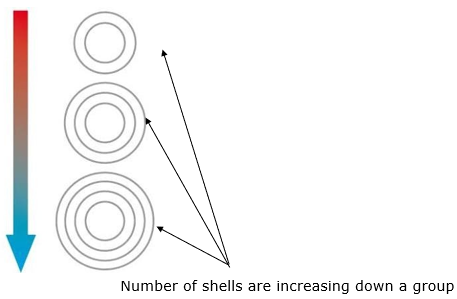b) Ionization energyIonization energy goes on increasing as we move from left to right because:⇒ The attraction of electrons towards the nucleus increases with increase in atomic number.⇒ Thus, the electrons are bonded tightly by the nucleus.⇒ It becomes difficult to remove electrons.⇒ Thus, ionization energy increases.Ionization energy goes on increasing as we move from top to bottom because:⇒ The outer electrons are adding in a new valence shell.⇒ Therefore, the distance between the outermost electron (valence electron) and the nucleus is also increasing⇒ It becomes easy to remove electrons.⇒ Thus, ionization energy decreases.c) Electron affinityElectron affinity goes on increasing as we move from left to right because:⇒ The nuclear charge increases from left to right so it easier to add an electron.⇒ Thus, it increases along a period.Electron affinity goes on decreasing as we move from top to bottom because:⇒ The size of the atom increases.⇒ The added electron becomes farther from the nucleus as we move⇒ Thus, it decreases as we down in a group.d) ElectronegativityElectronegativity increases along a period because-⇒ The atomic radius goes on decreasing as we move from left to right due to which the attraction between the outer electrons and nucleus increases.Electronegativity decreases along a group because-⇒ The atomic radius goes on increasing as we move from top to bottom due to which the attraction between the outer electrons and nucleus decreases.(b) a) The ionization energy increases as we move from left to right in a period. Thus, the ionization energy follows in the given order:Na < Al < Clb) The ionization energy increases as we move from left to right in a period. Thus, the ionization energy follows in the given order:Li < Be < Bc) The ionization energy increases as we move from left to right in a period. Thus, the ionization energy follows in the given order:C < N < Od) Ionization energy increases as we move from left to right in a period and decreases as we move down the group.By combining these two facts, the ionization energy follows in the given order:Na < F < Nee) Ionization energy decreases as we move down the group. Thus, the ionization energy follows in the given order:Be > Mg > CaQuestion 20.Name two elements that you would expect to have chemical properties similar to Mg. What is the basis for your choice?Answer:As we know that the elements in a group generally possess similar properties.Magnesium (Z=12) belongs to second group. So the two elements, calcium (Z=20) and beryllium (Z=4) have similar chemical properties like Mg because these elements also belong to second group.Question 21.On the basis of atomic number predict to which block the elements with atomic number 9, 37, 46 and 64 belongs to?Answer:Atomic number 9:The electronic configuration = 1s2 2s2 2p5The number of valence electrons = 7Period number = 7This means the element having atomic number 9 belongs to p-block.Atomic number 37:The electronic configuration = 1s2 2s2 2p6 3s2 3p6 3d10 4s2 4p6 5s1The number of valence electrons = 1Period number = 1This means the element having atomic number 37 belongs to s-block.Atomic number 46:The electronic configuration = [Kr]4d10The number of valence electrons = 10Period number = 10This means the element having atomic number 46 belongs to d-block.Atomic number 64:The electronic configuration = [Xe] 4f7 5d1 6s2The new electron enters into the f-orbital.This means the element having atomic number 64 belongs to f-block.Question 22.Using the periodic table, predict the formula of compound formed between and element X of group 13 and another element Y of group 16.Answer:To predict the formula of a compounds formed, first we will calculate the valency of each element.Element X of group 13Valency = 8-3 = 5Element Y of group 16Valency = 8-6 = 2The formula formed will be: X2Y5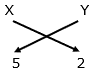Question 23.An element X belongs to 3rd period and group 2 of the periodic table. State(a) The no. of valence electrons(b) The valency(c) Whether it is metal or a nonmetal.Answer:Given: X belongs to 3rd period and second groupa) As the period number is three (2s2 2p1) thus, the number of valence electron = 1b) The valency is total number of electrons in the outermost shell. Thus, its valency is 1.c) X is a non-metalQuestion 24.An element has atomic number 19. Where would you expect this element in the periodic table and why?Answer:The element has atomic number = 19The electronic configuration = 1s2 2s2 2p6 3s2 3p6 4s1Number of valence electrons (4s1) = 1Period number = 1Group number = 4This means the element having atomic number 19 belongs to s-block because the period number is 1.Question 25.Aluminium does not react with water at room temperature but reacts with both dil. HCl and NaOH solution. Verify these statements experimentally. Write your observation with chemical equations. From these observations, can we conclude that A / is a metalloid?Answer:Aluminum with water:Aluminum does not get affected by water and does not react with it.Aluminum with dil.HClWhen aluminium is dissolved with hydrochloric acid (HCl), it gives aluminum chloride and hydrogen gas.2Al + 6HCl → 2AlCl3 + 3H2Aluminum with NaOHWhen aluminum is treated with NaOH, i.e., a base, it gives sodium aluminate and hydrogen gas.2Al + 2NaOH → 2NaAlO2 + H2Question 26.Collect the information about reactivity of VIII A group elements (noble gases) from intend or from your school library and prepare a report on their special character when compared to other elements of periodic table.Answer:i. Noble gases (group 18) are located in the far right of the periodic table.ii. All the orbitals in the valence shell of the noble gases are completely filled.iii. Noble gases neither have a tendency to lose or to gain electrons and hence do not enter into chemical combination.iv. Thus, they are earlier called “inert gases” as they are exhibit very low chemical reactivity.v. They have high ionization energies.vi. Noble gases have large positive electron gain enthalpies.vii. Boiling and melting points of noble gases are very low.viii. The noble gases are liquefied with great difficulty.ix. These gases are slightly soluble in water.Question 27.Collect information regarding metallic character of elements of 1A group and prepare report to support the idea of metallic character increases in a group as we move from top to bottom.Answer:i. As the elements of IA have low ionization energies, these metals have great tendency to lose their outermost electron and thus change into positive ions (cations).ii. These elements are therefore said to have strong electropositive character.iii. As ionization energy decreases on moving down the group, the electropositive character increases on moving down the group from lithium to caesium.Question 28.How do you appreciate the role of electronic configuration of the atoms of elements in periodic classification?Answer:Electronic configurationi. The characteristics of the groups and periods in the modern periodic table are because of the electronic configuration of the elements.ii. It is the electronic configuration of an element which decides the group and the period in which it is to be placed.iii. While going from top to bottom within any group, one electronic shell gets added at a time. From this we can say that the electronic configuration of the outermost shell is characteristic of a particular group.Question 29.Without knowing the electronic configuration of the atoms of elements Mendeleff still could arrange the element nearly close to the arrangements in the Modern periodic table. How can you appreciate this?Answer:Mendleeff’s periodic tablei. He arranged the elements in a systematic order of their increasing atomic masses.ii. He also discovered law which stated that “the physical and chemical properties of the elements are periodic function of their atomic masses.iii. There are eight vertical columns called as groups.iv. The horizontal rows in table called as periods.v. Based on the arrangement, he believed that some new elements would be discovered definitely.vi. He predicted the properties of these elements in advance.vii. After the elements were discovered, his predicted properties were almost the same as observed properties.viii. It was the extraordinary thinking of Mendleeff that made the chemist to accept the periodic table.Question 30.Comment on the position of hydrogen in periodic table.Answer:Position of hydrogeni. Hydrogen shows similarity with halogens (group VII). For example, the molecular formula of hydrogen is H2 while the molecular formulae of fluorine and chlorine are F and Cl2, respectively.ii. In the same way, there is a similarity in the chemical properties of hydrogen and alkali metals (group).iii. There is a similarity in the molecular formulae of the compounds of hydrogen alkali metals (Na, K, etc.) formed with chlorine and oxygen.iv. On considering the above properties, it cannot be decided whether the correct position of hydrogen is in the group of alkali metals (group I) or in the group of halogens (group VII).v. So to place the hydrogen, it was placed in group 1 without any proper conclusion.Question 31.How the position of elements in the periodic table helps you predict its chemical properties? Explain with an example.Answer:Position of elements in the periodic table gives a prediction of:i. Ionization energy of the element.ii. Electron affinity of the elementiii. Metallic and non-metallic properties.iv. ElectronegativityFor example: An element having atomic number = 9Its group number is 2 and period number is 2.This means the element belongs to non-metals.

PDF FILE TO YOUR EMAIL IMMEDIATELY PURCHASE NOTES & PAPER SOLUTION. @ Rs. 50/- each (GST extra)

HINDI ENTIRE PAPER SOLUTION

MARATHI PAPER SOLUTION

SSC MATHS I PAPER SOLUTION

SSC MATHS II PAPER SOLUTION

SSC SCIENCE I PAPER SOLUTION

SSC SCIENCE II PAPER SOLUTION

SSC ENGLISH PAPER SOLUTION

SSC & HSC ENGLISH WRITING SKILL

HSC ACCOUNTS NOTES

HSC OCM NOTES

HSC ECONOMICS NOTES

HSC SECRETARIAL PRACTICE NOTES

# 2019 Board Paper Solution

HSC ENGLISH SET A 2019 21st February, 2019

HSC ENGLISH SET B 2019 21st February, 2019

HSC ENGLISH SET C 2019 21st February, 2019

HSC ENGLISH SET D 2019 21st February, 2019

SECRETARIAL PRACTICE (S.P) 2019 25th February, 2019

HSC XII PHYSICS 2019 25th February, 2019

CHEMISTRY XII HSC SOLUTION 27th, February, 2019

OCM PAPER SOLUTION 2019 27th, February, 2019

HSC MATHS PAPER SOLUTION COMMERCE, 2nd March, 2019

HSC MATHS PAPER SOLUTION SCIENCE 2nd, March, 2019

SSC ENGLISH STD 10 5TH MARCH, 2019.

HSC XII ACCOUNTS 2019 6th March, 2019

HSC XII BIOLOGY 2019 6TH March, 2019

HSC XII ECONOMICS 9Th March 2019

SSC Maths I March 2019 Solution 10th Standard11th, March, 2019

SSC MATHS II MARCH 2019 SOLUTION 10TH STD.13th March, 2019

SSC SCIENCE I MARCH 2019 SOLUTION 10TH STD. 15th March, 2019.

SSC SCIENCE II MARCH 2019 SOLUTION 10TH STD. 18th March, 2019.

SSC SOCIAL SCIENCE I MARCH 2019 SOLUTION20th March, 2019

SSC SOCIAL SCIENCE II MARCH 2019 SOLUTION, 22nd March, 2019

XII CBSE - BOARD - MARCH - 2019 ENGLISH - QP + SOLUTIONS, 2nd March, 2019

# HSCMaharashtraBoardPapers2020

(Std 12th English Medium)

HSC ECONOMICS MARCH 2020

HSC OCM MARCH 2020

HSC ACCOUNTS MARCH 2020

HSC S.P. MARCH 2020

HSC ENGLISH MARCH 2020

HSC HINDI MARCH 2020

HSC MARATHI MARCH 2020

HSC MATHS MARCH 2020

# SSCMaharashtraBoardPapers2020

(Std 10th English Medium)

English MARCH 2020

HindI MARCH 2020

Hindi (Composite) MARCH 2020

Marathi MARCH 2020

Mathematics (Paper 1) MARCH 2020

Mathematics (Paper 2) MARCH 2020

Sanskrit MARCH 2020

Sanskrit (Composite) MARCH 2020

Science (Paper 1) MARCH 2020

Science (Paper 2)

Geography Model Set 1 2020-2021

MUST REMEMBER THINGS on the day of Exam

Are you prepared? for English Grammar in Board Exam.

Paper Presentation In Board Exam

How to Score Good Marks in SSC Board Exams

Tips To Score More Than 90% Marks In 12th Board Exam

How to write English exams?

How to prepare for board exam when less time is left

How to memorise what you learn for board exam

No. 1 Simple Hack, you can try out, in preparing for Board Exam

How to Study for CBSE Class 10 Board Exams Subject Wise Tips?

JEE Main 2020 Registration Process – Exam Pattern & Important Dates

NEET UG 2020 Registration Process Exam Pattern & Important Dates

How can One Prepare for two Competitive Exams at the same time?

8 Proven Tips to Handle Anxiety before Exams!